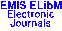PUBLICATIONS DE L'INSTITUT MATHÉMATIQUE (BEOGRAD) (N.S.) Vol. 70(84), pp. 26--36 (2001)

Previous Article

Next Article

Contents of this Issue

Other Issues

ELibM Journals

ELibM Home

EMIS Home

## Orthogonal polynomials and regularly varying sequences

### Slavko Simi\'c

Matemati\v cki institut SANU, Kneza Mihaila 35, 11000 Beograd, p.p. 367, Yugoslavia

Abstract: We introduce a method of estimating asymptotic behaviour of polynomials $Q_n^{(\alpha)}(x):=\sum_{k\le n}c_k a_{nk} x^k$, $n\to\infty$, related to a given polynomial $Q_n(x):=\sum_{k\le n} a_{nk}x^k$, where $(c_k)$, $k\in N$ is any regularly varying sequence of index $\alpha$ in the sense of Karamata. Then we apply our results to classical orthogonal polynomials as relevant examples.

Classification (MSC2000): 42C05; 26A12

Full text of the article: